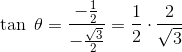Scan QR code or get instant email to install app

Question:

# 1212If \u03B8 is an obtuse angle andin Quadrant III, what is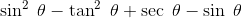?

A 1212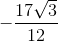explanation

1212Use the trigonometric identity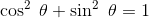to solve for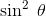, then use the Quadrant information to solve for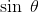, which can then be used to find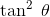and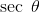.
Substitute the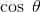into the trigonometric identity: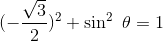, which becomes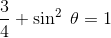, so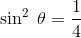.
Evaluate the square root to find sin\u03B8: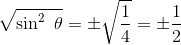. It is given that the cosine value is in Quadrant III, so the sine value is negative and equals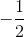.
Recall that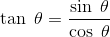and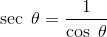. Substitute the appropriate values to find tangent and secant: